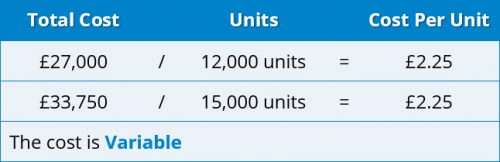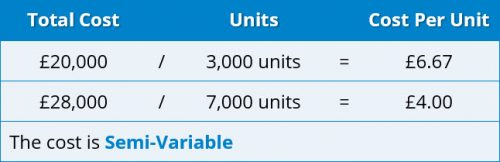# Getting to Grips with AAT Cost Behaviour## Is AAT Cost Behaviour a Challenge for You?

There are some topics included within the AAT syllabus that are so important that they are assessed at all levels of the AAT qualification.  The accounting equation is one such topic, and another is the subject of this blog, cost behaviour.  Despite its importance, students at all levels are frequently confused by cost behaviour and make fundamental mistakes in their calculations.  In this blog I will explain the basics of cost behaviour and how you might use your understanding in calculations.

#### What do we mean by cost behaviour?

Cost behaviour refers to the way costs will change when levels of production change within in an organisation.  At Level 2 (Foundation Certificate in Accounting) students need to know about three kinds of cost behaviour:

• Variable costs
• Fixed costs
• Semi-variable costs

In addition, for Level 3 (Advanced Diploma in Accounting) and Level 4 (Professional Diploma in Accounting), students also need to understand the behaviour of stepped fixed costs.

Let’s look at each cost in more detail.

#### Variable costs

As its name suggests, a variable cost is a cost which varies or changes with production levels. Using a domestic example, if you are cooking Sunday lunch for 8 people you will need more potatoes than if you are cooking lunch for 2 people.   The more you make, the higher the total cost.  There is an important point here, which students frequently fail to recognise properly.  This is that the variable cost per unit stays the same, while the total cost increases or decreases with production.  Using the Sunday lunch example again, if you buy potatoes for 8 people you will pay the same amount per kilogram as if you are buying potatoes for 2 people.

You can use this information to work out the variable cost per unit of an item.  For example, if you learn that the total cost of materials is £22,500 for 5,000 units, divide the cost by the units to get a variable cost per unit of £4.50.  You will use this calculation frequently in budgeting questions.

You may be told that total costs at 12,000 units are £27,000 and at 15,000, total costs are £33,750.  Is this a variable cost?  The way to check is to divide £27,000 by 12,000 giving a cost per unit of £2.25.   Now divide £33,750 by 15,000 units.  The cost per unit is the same amount i.e. £2.25.  As we saw above, where the total cost changes but the cost per unit is the same, the cost is a variable cost, so we can say that this example is a variable cost.#### Fixed costs

This kind of cost can confuse students because a fixed cost is not a static, unmoving, cost.  Rather, it is a cost that is not directly affected by levels of production.  Suppose you rent a workshop to make your product.  Once you have signed the lease, you will have to pay the rent whether you make any units or not.  You pay rent for the month, the quarter or the year, not according to the number of units you make.  Thus a fixed cost is a cost that does not relate to production levels, but to a period of time.  In most circumstances this is all you need to know about fixed costs, but bear in mind that costs are fixed only over a range of activity.  For example, the workshop referred to above may be large enough to make up to 10,000 units a year.  The range of activity for the fixed cost is 0-10,000 units.  If you want to make more than 10,000 units you will need bigger premises so your rental cost will change, presumably increasing.

Fixed costs work in the opposite way to variable costs in that the total cost stays the same but the cost per unit will increase or decrease according to production.  A fixed cost of £15,000 will be the total fixed cost at production levels of 3,000 units or 5,000 units.  However, if we divide the £15,000 by 3,000 units we get a cost per unit of £5 and if we divide £15,000 by 5,000 we get a fixed cost per unit of £3.  The fixed cost per unit decreases as production levels increase, as the total cost can be split between more units.A question might give you this information:

At 6,000 units this cost is £4.20 and at 9,000 units it is £2.80 per unit

As the costs per unit at the two levels of production are not the same we can state that this is not a variable cost, but is it a fixed cost?

• 6,000 x £4.20 = £25,200
• 9,000 x £2.80 = £25,200

As the total cost at both levels is the same amount, we can see that this is indeed a fixed cost.

#### Semi-variable costs

A semi-variable cost (or a semi-fixed cost) is a cost that includes both a fixed element and a variable element.  Although not relevant for production, a taxi fare is a good example of a semi-variable cost.   As you get into the taxi you can see a charge already displayed on the meter.  This is the fixed element of the cost.  Once you set off, the fare starts to increase rapidly until you get to your destination.  This is the variable element of the cost.

You can use the high-low technique to separate the fixed and variable elements of the total cost.  This is fully explained in your textbooks and learning materials so will not be covered here.

Unlike the fixed cost and variable costs, both total cost and cost per unit will change with different activity levels.

Suppose you are told that at 3,000 units the total cost is £20,000 and at 7,000 units the cost is £28,000.  We have seen above that a fixed cost has the same total cost at different production levels.  Here the total cost is different at the two production levels so we know this is not a fixed cost, but is it a variable cost?

If it is a variable cost, the cost per unit will be the same at both levels. If we divide £20,000 by 3,000 we get a cost of £6.67 (rounded) per unit.  Dividing £28,000 by 7,000 the cost per unit is £4.  Since both total cost and cost per unit are different at the two activity levels, this must be a semi-variable cost.#### Stepped cost

This is a type of fixed cost.  The cost remains the same up to a certain activity level, then increases suddenly and remains the same at the new activity level.  In other words, the cost increases in steps.  An example of this would be the cost of salaries for production supervisors, where an additional supervisor is needed for every 10 staff.  While production staff number 1-10, only one supervisor will be needed but as soon as the 11th production worker is recruited, a second supervisor will be needed.

It is common in exam questions for students to have to calculate a total stepped cost by working out the number of steps.

Let us suppose that a leased production machine can produce 5,000 units at a cost of £6,000 per machine.  What would be the lease cost of producing 28,000 units?

We can see that 28,000 divided by 5,000 gives a figure of 5.6.  This tells us the number of ‘steps’ or, (in this case), machines that are needed.  Remember that you must use whole numbers to work out the steps.  Here, we would need 6 machines.  If we had 5 machines, we could make 25,000 units but we need to make 28,000 so a 6th machine is needed.  Students often forget to round their answers up in this way, so be careful in calculating the number of steps.#### Conclusion

Cost behaviour is a fundamentally important topic for management accounting and will continue to be assessed at all levels of the AAT qualifications.

You will have noticed that this blog includes many calculations of cost per unit.  When dealing with cost behaviour questions, it is essential to calculate both cost per unit and total cost to enable you to work out the type of cost correctly.  Students sometimes seem reluctant to calculate the cost per unit figure and make mistakes in their answers as a result.  It is worth spending time to ensure you thoroughly understand the way that the different types of cost behave – every AAT student should be able to cope with any question on this important topic.

##### Accountancy Learning

Accountancy Learning Ltd specialises in the provision for accountancy training. We offer a wide spectrum of courses in accountancy and bookkeeping from beginner's level to the full AAT Accounting Technician qualification centered around our Virtual Learning Environment, Moodle. We also provide impartial advice on progression options to ACA, ACCA, CIMA, and ATT.